Home / Electrical Circuits / Electric Current, Voltage, and Resistance Overview | Three Basic Electrical Quantities

# Electric Current, Voltage, and Resistance Overview | Three Basic Electrical Quantities

Want create site? Find Free WordPress Themes and plugins.

Electric Current, voltage, and resistance are three of the fundamental electrical properties. Stated simply,

current: is the directed flow of charge through a conductor.
Voltage: is the force that generates the current.
Resistance: is an opposition to current that is provided by the material, component, or circuit.

Electric Current, Voltage, and resistance are the three primary properties of an electrical circuit. The relationships among them are defined by the fundamental law of circuit operation, called Ohm’s law.

## Electric Current

As you know, an outside force can break an electron free from its parent atom. In copper (and other metals), very little external force is required to generate free electrons. In fact the thermal energy (heat) present at room temperature (220C) can generate free electrons. The number of electrons generated varies directly with temperature. In other words, higher temperatures generate more free electrons.

The motion of the free electrons in copper is random when no directing force is applied. That is, the free electrons in copper are random when no directing force is applied. That is, the free electrons move in every direction, as shown in Figure 1. Since, the free electrons are moving in every direction, this net flow of electrons in any direction is zero.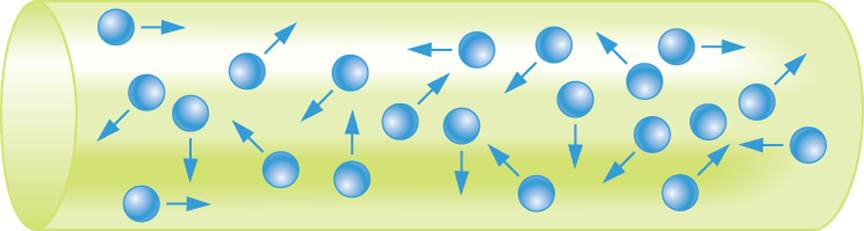Figure 1 Random electron motion in copper

Figure 2 Illustrates what happens when an external force causes all of the electrons to move in the same direction. In this case, a negative potential is applied to one end of the copper and a positive potential is applied to the other. As a result, the free electrons all move from negative to positive, and we can say that we have a directed flow of charge (electrons). This directed flow of electrons is called electric current.Figure 2 Directed electron motion in copper.

Let’s look at what happens on a larger scale when electron motion is directed by an outside force. In Figure 3, the negative potential directs electron flow (current) toward the positive potential. The current passes through the lamp, causing it to produce light and heat. The more intense the current (meaning the greater its value), the greater the light and heat produced by the bulb.Figure 3 Current through a basic lamp circuit.

Electric Current is represented in formulas by the letter I (For intensity). The intensity of current is determined by the amount of charge flowing per second. The greater the flow of charge per second, the more intense the current.

Coulombs and Amperes

The change on a single electron is not sufficient to provide in a practical unit of measure for charge. Therefore, the Coulomb (C) is used as the basic unit of charge One coulomb equals the total charge on 6.25 × 1018 electrons. When, one coulomb of charge passes a point in one second, we have one Ampere (A) of electric current. In other words,

$\begin{matrix}1\text{ ampere=1 Coulomb per second} \\Or \\1\text{ A=1 C/s} \\\end{matrix}$

The total current passing a point (in amperes) can be found by dividing the total charge ( in coulombs) by the time ( in seconds) . By Formula

$\begin{matrix}I=\frac{Q}{t} & {} & \left( 1 \right) \\\end{matrix}$

Where

I= the intensity of electric current in amperes

Q= the total charge, in coulombs

T= the time it takes the charge to pass a point, in seconds

This relationship is illustrated in Example 1.

Example 1

Three coulombs of charge pass through a copper wire every second. What is the value of electric current?

Solution

Using equation 1, the current is found as

$I=\frac{Q}{t}=\frac{3C}{1s}=3{}^{C}/{}_{s}=3A$

Example 1 is included here to help you understand the relationship between amperes, coulombs, and seconds. In practice, electric current is not calculated using Equation 1 because you cannot directly measure coulombs in charge. As you will learn, there are far more practical ways to calculate current.

Two Theories: Conventional Current and Electron Flow.

There are two theories that describe electric current, and you will come across both in practice.

The Conventional Current theory defines current as the flow of charge from positive to negative. This theory is called “conventional current” because it is the older of the two approaches to current, and for many years was the only one taught outside of military and trade schools.

Electron Flow is the newer of the two current theories. Electron flow theory defines current as the flow of electrons from negative to positive.

The two electric current theories are contrasted in Figure 4. Each circuit contains a battery and a lamp. Conventional current begins at the positive battery terminal, passes through the lap, and returns to the battery through its negative terminal. Electron flow is in the opposite direction: It begins at the negative terminal, passes through the lamp, and returns to the battery through its positive terminal.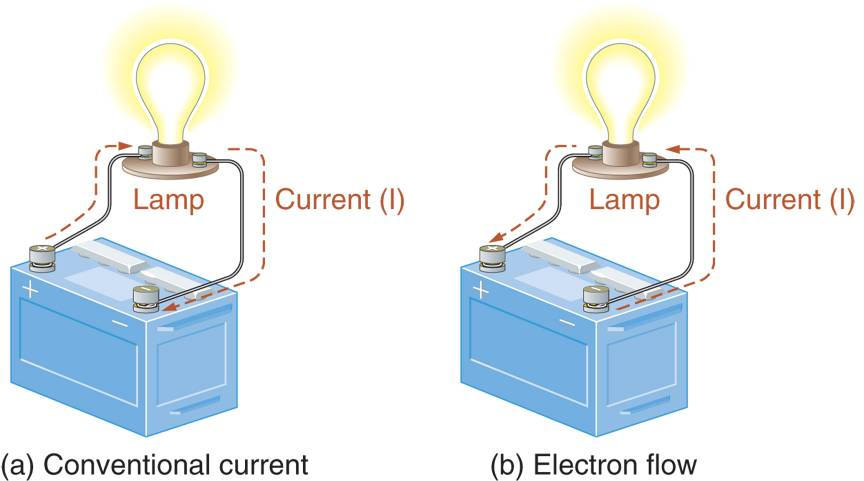Figure 4 Conventional current and electron flow.

It is worth nothing that the two circuits in Figure 4 are identical. The only difference between the two is how we describe the electric current. In practice, how you view current does not affect any circuit calculations, measurements, or test procedures. Even so you should get comfortable with both viewpoints, since both are used by many engineers technicians, and technical publications. In this text, we take the electron flow approach to current. That is, we will assume current is the flow of electrons from negative to positive.

Direct Current (DC) Versus Alternating Current (AC)

Current is generally classified as being either Direct Current (DC) or Alternating Current (AC). The differences between direct current and alternating current are illustrated in Figure 5.Figure 5 Direct current (DC) and alternating current (AC).

Direct current is unidirectional. That is, the flow of charge is always in the same direction. The term direct current usually implies that the current has a fixed value. For example, the graph in Figure 5a shows that the current has a constant value of 1A. While a fixed value is implied, direct current can change in value. However, the direction of current does not change.

Alternating Current is Bidirectional. That is, the direction of current changed continually. For example, in figure 5b, the graph shows that the current builds to a peak value in one direction and then builds to a peak value in the other direction. Note that the alternating current represented by the graph not only changes direction but is constantly changing in value.

Electric Current Produces Heat

Whenever electric current is generated through a component or circuit, heat is produced. The amount of heat varies with the level of current: The greater the current, the more heat it produces. This is why many high-current components, like motors, get hot when they are operated. Some High-current circuits get so hot that they have to be cooled.

The heat produced by electric current is sometimes a desirable thing. Toasters, electric stoves, and heat lamps are common items that take advantage of the heat produced by current.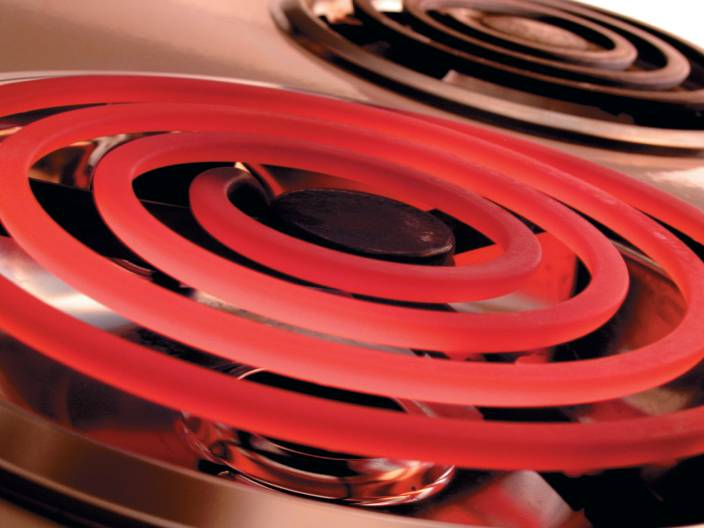Figure 6 High current causes a stove heating element burner to glow red.

Putting it all together

Free electrons are generated in copper at room temperature. When undirected, the motion of these free electrons is random, and the net flow of electrons in any one direction is zero.

When directed by an outside force, free electrons are forced to move in a uniform direction. This directed flow of charge is referred to as electric current.

Electric Current is represented by the letter I, which stands for intensity. The intensity of current depends on the amount of charge moved and the time required to move it.

Electric Current is measured in amperes (A). When one coulomb of current passes a point every second, you have one ampere of current.

There are two current theories. The electron flow theory describes current as the flow of charge (electrons) from negative to positive. The conventional current theory describes current as the flow of charge from positive to negative. Both approaches are widely followed. The way you view current does not affect the outcome of any circuit calculations, measurements, or test procedures.

Most electrical and electronic systems contain both direct current (DC) and alternating current (AC) circuits. In DC circuits, the current is always in the same direction. In AC circuits, current continually changes direction.

Review Questions

How are free electrons generated in a conductor at room temperature?

The thermal energy (heat) present at room temperature is enough to generate free electrons.

What is electric current? What factors affect the intensity of electric current?

Current is the directed flow of electrons in a material. The intensity of current depends on the amount of charge moved and the time required to move it.

What is a coulomb?

One coulomb equals the total charge on 6.25 × 1018 electrons.

What is he basic unit of electric current?

The ampere is the basic unit of electric current. It is defined as 1 coulomb per second or 1 A = 1 C/s.

Contrast the electron flow and convention current theories.

Conventional current theory defines current as the flow of charge from positive to negative. Electron flow is the flow of charge from negative to positive.

## Voltage

Voltage can be described as a force that generates the flow of electrons (current) through a circuit. In this section, we take a detailed look at voltage and how it generates current.

Generating Current with a Battery

Battery in Figure 7a has two terminals. The positive (+) terminal has an excess of positive ions and is described as having a positive potential. The negative (-) terminal has an excess of electrons and is described as having a negative potential.Figure 7 A difference of potential and a resulting current.

Thus there is a Difference of Potential, or voltage (V), between the two terminals.

If we connect the two terminals of the battery with the copper wire and lamp (Figure7b), a current is produced as the electrons are drawn to the positive terminal of the battery. In other words, there is a directed flow of electrons from the negative (-) terminal to the positive (+) terminal of the battery.

There are several important points that need to be made:

1. Voltage is a force that moves electrons, for this reason, it is often referred to as Electrical Force (E) or Electromotive Force (EMF).

2. Current and voltage is not the same thing. Current is the directed flow of electrons from negative to positive. Voltage is the electrical force that generates current. In other words, current occurs as a result of an applied voltage (electric force).

The volt (V) is the unit of measure for voltage. Technically defined, one volt is the amount of electrical force that uses one joule (J) of energy to move one coulomb (C) of charge that is,

\begin{align}& \text{1 volt= 1 joule per coulomb} \\& \text{or} \\& \text{1 V=1 }{}^{J}/{}_{C} \\\end{align}

Review Questions

What is voltage?

Voltage is the force that generates current in a circuit.

How does voltage generate a current through a wire?

A voltage source has an excess of electrons (negative charge) on one terminal and an excess of positive ions on the other. This is referred to as a potential difference. The excess electrons at the negative terminal are attracted by the positive ions on the positive terminal. This results in the flow of charge in any wire that connects the two terminals of the voltage source.

What is the unit of measure for voltage? How is it defined?

The unit of measure for voltage is the volt. One volt is the amount of electrical force that uses one joule (J) of energy to move one coulomb (C) of charge. 1 V = 1 J/C.

How would you define a coulomb in terms of voltage and energy?

1 coulomb equals 1 joule per volt, 1 C = 1 J/V

How would you define a joule in terms of voltage and charge?

1 joule equals 1 V times 1 coulomb, 1 J = 1 V × 1 C

## Resistance

All elements provide some opposition to current. This opposition to current is called resistance. The higher the resistance of an element, component, or circuit, the lower the current produced by the given voltage.

Resistance (R) is measured in Ohms. Ohms are represented using the Greek letter omega (Ω). Technically defined, one ohm is the amount of resistance that limits current to one ampere when one volt of electrical force is applied. This definition is illustrated in Figure 8.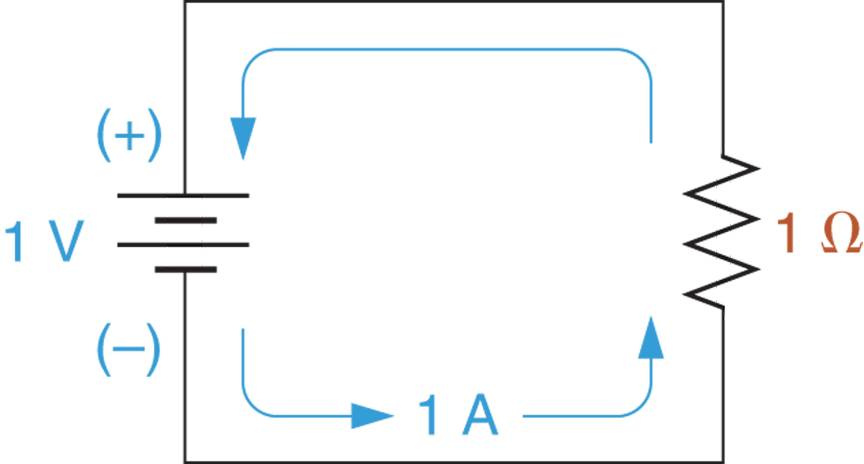Figure 8 A basic electric circuit.

The schematic diagram in Figure 8 shows a battery that is connected to a resistor. A resistor is a component that provides a specific amount of resistance. As shown in the figure, a resistance of 1Ω limits the current to 1A when 1V is applied. Note that the long end-bar on the battery schematic symbol represents the battery’s positive terminal and the short end bar represents its Negative terminal.

Putting It All Together

We have now defined charge, current, voltage and resistance. For convenience, these electrical properties are summarized in Table 1.

Table 1: Basic Electrical Properties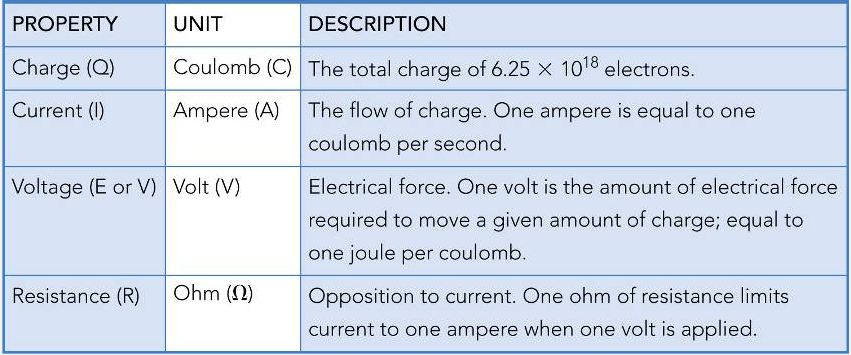Many of the properties listed in Table 1 can be defined in terms of the others. For example, in our discussion on resistance, we said that one ohm is the amount of resistance that limits current to one amp when one volt of electrical force is applied. By the same token, we can redefine the ampere and the volt as follows:

1. One ampere is the amount of current that is generated when one volt of electrical force is applied to one ohm of resistance.
2. One volt is the amount of electrical force required to generate one amp of current through one
ohm of resistance.

Review Question

What is resistance?

Resistance is the opposition to current.

What is the basic unit of resistance and how is it defined?

The unit of resistance is the Ohm (Ω). One ohm is the amount of resistance that limits current to 1 ampere when one volt is applied. 1 Ω = 1 A/V.

Define each of the following values in terms of the other two: current, voltage, and resistance.

1 V is the force required to cause 1 ampere of current through 1 ohm of resistance.
1 A is the current that results from when 1 volt is applied to 1 ohm of resistance.
1 Ω is the resistance that limits current to 1 ampere when 1 volt is applied.

Did you find apk for android? You can find new Free Android Games and apps.